$$\newcommand{\id}{\mathrm{id}}$$ $$\newcommand{\Span}{\mathrm{span}}$$ $$\newcommand{\kernel}{\mathrm{null}\,}$$ $$\newcommand{\range}{\mathrm{range}\,}$$ $$\newcommand{\RealPart}{\mathrm{Re}}$$ $$\newcommand{\ImaginaryPart}{\mathrm{Im}}$$ $$\newcommand{\Argument}{\mathrm{Arg}}$$ $$\newcommand{\norm}{\| #1 \|}$$ $$\newcommand{\inner}{\langle #1, #2 \rangle}$$ $$\newcommand{\Span}{\mathrm{span}}$$

# 2.7: Introduction to Inequalities and Interval Notation

$$\newcommand{\vecs}{\overset { \rightharpoonup} {\mathbf{#1}} }$$ $$\newcommand{\vecd}{\overset{-\!-\!\rightharpoonup}{\vphantom{a}\smash {#1}}}$$$$\newcommand{\id}{\mathrm{id}}$$ $$\newcommand{\Span}{\mathrm{span}}$$ $$\newcommand{\kernel}{\mathrm{null}\,}$$ $$\newcommand{\range}{\mathrm{range}\,}$$ $$\newcommand{\RealPart}{\mathrm{Re}}$$ $$\newcommand{\ImaginaryPart}{\mathrm{Im}}$$ $$\newcommand{\Argument}{\mathrm{Arg}}$$ $$\newcommand{\norm}{\| #1 \|}$$ $$\newcommand{\inner}{\langle #1, #2 \rangle}$$ $$\newcommand{\Span}{\mathrm{span}}$$ $$\newcommand{\id}{\mathrm{id}}$$ $$\newcommand{\Span}{\mathrm{span}}$$ $$\newcommand{\kernel}{\mathrm{null}\,}$$ $$\newcommand{\range}{\mathrm{range}\,}$$ $$\newcommand{\RealPart}{\mathrm{Re}}$$ $$\newcommand{\ImaginaryPart}{\mathrm{Im}}$$ $$\newcommand{\Argument}{\mathrm{Arg}}$$ $$\newcommand{\norm}{\| #1 \|}$$ $$\newcommand{\inner}{\langle #1, #2 \rangle}$$ $$\newcommand{\Span}{\mathrm{span}}$$

Learning Objectives

• Graph the solutions of a single inequality on a number line and express the solutions using interval notation.
• Graph the solutions of a compound inequality on a number line, and express the solutions using interval notation.

## Unbounded Intervals

An algebraic inequality, such as $$x≥2$$, is read “$$x$$ is greater than or equal to $$2$$.” This inequality has infinitely many solutions for $$x$$. Some of the solutions are $$2, 3, 3.5, 5, 20,$$ and $$20.001$$. Since it is impossible to list all of the solutions, a system is needed that allows a clear communication of this infinite set. Two common ways of expressing solutions to an inequality are by graphing them on a number line and using interval notation.

To express the solution graphically, draw a number line and shade in all the values that are solutions to the inequality. Interval notation is textual and uses specific notation as follows:Figure 2.7.1

Determine the interval notation after graphing the solution set on a number line. The numbers in interval notation should be written in the same order as they appear on the number line, with smaller numbers in the set appearing first. In this example, there is an inclusive inequality, which means that the lower-bound 2 is included in the solution. Denote this with a closed dot on the number line and a square bracket in interval notation. The symbol (∞) is read as infinity and indicates that the set is unbounded to the right on a number line. Interval notation requires a parenthesis to enclose infinity. The square bracket indicates the boundary is included in the solution. The parentheses indicate the boundary is not included. Infinity is an upper bound to the real numbers, but is not itself a real number: it cannot be included in the solution set.

Now compare the interval notation in the previous example to that of the strict, or noninclusive, inequality that follows:Figure 2.7.2

Strict inequalities imply that solutions may get very close to the boundary point, in this case 2, but not actually include it. Denote this idea with an open dot on the number line and a round parenthesis in interval notation.

Example $$\PageIndex{1}$$

Graph and give the interval notation equivalent:

$$x<3$$.

Solution:

Use an open dot at $$3$$ and shade all real numbers strictly less than $$3$$. Use negative infinity $$(−∞)$$ to indicate that the solution set is unbounded to the left on a number line.Figure 2.7.3

Interval notation: $$(-∞, 3)$$

Example $$\PageIndex{2}$$

Graph and give the interval notation equivalent:

$$x≤5$$.

Solution:

Use a closed dot and shade all numbers less than and including 5.Figure 2.7.4

Interval notation: $$(−∞, 5]$$

It is important to see that $$5≥x$$ is the same as $$x≤5$$. Both require values of $$x$$ to be smaller than or equal to $$5$$. To avoid confusion, it is good practice to rewrite all inequalities with the variable on the left. Also, when using text, use “inf” as a shortened form of infinity. For example, $$(−∞, 5]$$ can be expressed textually as $$(−$$inf, $$5]$$.

A compound inequality is actually two or more inequalities in one statement joined by the word “and” or by the word “or.” Compound inequalities with the logical “or” require that either condition must be satisfied. Therefore, the solution set of this type of compound inequality consists of all the elements of the solution sets of each inequality. When we join these individual solution sets it is called the union, denoted $$∪$$. For example, the solutions to the compound inequality $$x<3$$ or $$x≥6$$ can be graphed as follows:Figure 2.7.5

Sometimes we encounter compound inequalities where the separate solution sets overlap. In the case where the compound inequality contains the word “or,” we combine all the elements of both sets to create one set containing all the elements of each.

Example $$\PageIndex{3}$$

Graph and give the interval notation equivalent:

$$x≤−1$$ or $$x<3$$.

Solution:

Combine all solutions of both inequalities. The solutions to each inequality are sketched above the number line as a means to determine the union, which is graphed on the number line below.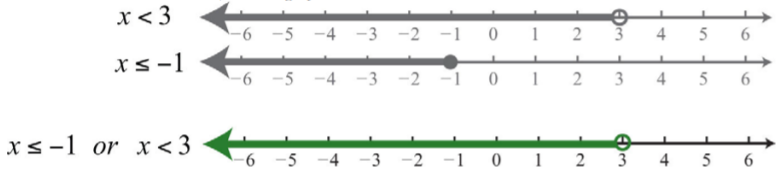Figure 2.7.6

Interval notation: $$(−∞, 3)$$

Any real number less than $$3$$ in the shaded region on the number line will satisfy at least one of the two given inequalities.

Example $$\PageIndex{4}$$

Graph and give the interval notation equivalent:

$$x<3$$ or $$x≥−1$$.

Solution:

Both solution sets are graphed above the union, which is graphed below.Figure 2.7.7

Interval notation: $$R = (−∞, ∞)$$

When you combine both solution sets and form the union, you can see that all real numbers satisfy the original compound inequality.

In summary,Figure 2.7.8Figure 2.7.9

andFigure 2.7.10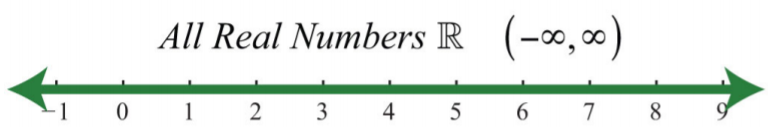Figure 2.7.11

## Bounded Intervals

An inequality such as

$$-1\leq x<3$$

reads “$$−1$$ one is less than or equal to $$x$$ and $$x$$ is less than three.” This is a compound inequality because it can be decomposed as follows:

$$-1\leq x$$ and $$x<3$$

The logical “and” requires that both conditions must be true. Both inequalities are satisfied by all the elements in the intersection, denoted $$∩$$, of the solution sets of each.

Example $$\PageIndex{5}$$

Graph and give the interval notation equivalent:

$$x<3$$ and $$x≥−1$$.

Solution:

Determine the intersection, or overlap, of the two solution sets. The solutions to each inequality are sketched above the number line as a means to determine the intersection, which is graphed on the number line below.Figure 2.7.12

Here $$x=3$$ is not a solution because it solves only one of the inequalities.

Interval notation: $$[−1, 3)$$

Alternatively, we may interpret $$−1≤x<3$$ as all possible values for $$x$$ between or bounded by $$−1$$ and $$3$$ on a number line. For example, one such solution is $$x=1$$. Notice that $$1$$ is between $$−1$$ and $$3$$ on a number line, or that $$−1 < 1 < 3$$. Similarly, we can see that other possible solutions are $$−1, −0.99, 0, 0.0056, 1.8$$, and $$2.99$$. Since there are infinitely many real numbers between $$−1$$ and $$3$$, we must express the solution graphically and/or with interval notation, in this case $$[−1, 3)$$.

Example $$\PageIndex{6}$$

Graph and give the interval notation equivalent:

$$−\frac{3}{2}<x<2$$.

Solution:

Shade all real numbers bounded by, or strictly between, $$−\frac{3}{2}=−1\frac{1}{2}$$ and $$2$$.Figure 2.7.13

Interval notation: $$(−\frac{3}{2}, 2)$$

Example $$\PageIndex{7}$$

Graph and give the interval notation equivalent:

$$−5<x\leq 15$$

Solution:

Shade all real numbers between $$−5$$ and $$15$$, and indicate that the upper bound, $$15$$, is included in the solution set by using a closed dot.Figure 2.7.14

Interval notation: $$(−5, 15]$$

In the previous two examples, we did not decompose the inequalities; instead we chose to think of all real numbers between the two given bounds.

In summary,Figure 2.7.15

## Set-Builder Notation

In this text, we use interval notation. However, other resources that you are likely to encounter use an alternate method for describing sets called set-builder notation. We have used set notation to list the elements such as the integers

$$\{...,-3,-2,-1,0,1,2,3,...\}$$

The braces group the elements of the set and the ellipsis marks indicate that the integers continue forever. In this section, we wish to describe intervals of real numbers—for example, the real numbers greater than or equal to $$2$$.Figure 2.7.16

Since the set is too large to list, set-builder notation allows us to describe it using familiar mathematical notation. An example of set-builder notation follows:

$$\{ x∈\mathbb{R} | x\geq 2\}$$

Here $$x∈R$$ describes the type of number, where the symbol $$(∈)$$ is read “element of.” This implies that the variable $$x$$ represents a real number. The vertical bar $$(|)$$ is read “such that.” Finally, the statement $$x≥2$$ is the condition that describes the set using mathematical notation. At this point in our study of algebra, it is assumed that all variables represent real numbers. For this reason, you can omit the “$$∈R$$” and write $$\{x|x≥2\}$$, which is read “the set of all real numbers $$x$$ such that $$x$$ is greater than or equal to $$2$$.”Figure 2.7.17

To describe compound inequalities such as $$x<3$$ or $$x≥6$$, write $$\{x|x<3$$ or $$x≥6\}$$, which is read “the set of all real numbers $$x$$ such that $$x$$ is less than $$3$$ or $$x$$ is greater than or equal to $$6$$.”Figure 2.7.18

Write bounded intervals, such as $$−1≤x<3$$, as $$\{x|−1≤x<3\}$$, which is read “the set of all real numbers $$x$$ such that $$x$$ is greater than or equal to $$−1$$ and less than $$3$$.”

## Key Takeaways

• Inequalities usually have infinitely many solutions, so rather than presenting an impossibly large list, we present such solutions sets either graphically on a number line or textually using interval notation.
• Inclusive inequalities with the “or equal to” component are indicated with a closed dot on the number line and with a square bracket using interval notation.
• Strict inequalities without the “or equal to” component are indicated with an open dot on the number line and a parenthesis using interval notation.
• Compound inequalities that make use of the logical “or” are solved by solutions of either inequality. The solution set is the union of each individual solution set.
• Compound inequalities that make use of the logical “and” require that all inequalities are solved by a single solution. The solution set is the intersection of each individual solution set.
• Compound inequalities of the form $$n<A<m$$ can be decomposed into two inequalities using the logical “and.” However, it is just as valid to consider the argument $$A$$ to be bounded between the values $$n$$ and $$m$$.

Exercise $$\PageIndex{1}$$ Simple Inequalities

Graph all solutions on a number line and provide the corresponding interval notation.

1. $$x≤10$$
2. $$x>−5$$
3. $$x>0$$
4. $$x≤0$$
5. $$x≤−3$$
6. $$x≥−1$$
7. $$−4<x$$
8. $$1≥x$$
9. $$x<−\frac{1}{2}$$
10. $$x≥−\frac{3}{2}$$
11. $$x≥−1\frac{3}{4}$$
12. $$x<\frac{3}{4}$$

1. $$(−∞, 10]$$Figure 2.7.19

3. $$(0, ∞)$$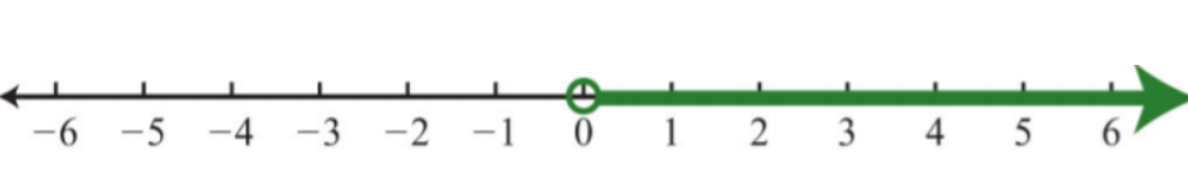Figure 2.7.20

5. $$(−∞, −3]$$Figure 2.7.21

7. $$(−4, ∞)$$Figure 2.7.22

9. $$(−∞, −\frac{1}{2})$$Figure 2.7.23

11. $$[−1\frac{3}{4}, ∞)$$Figure 2.7.24

Exercise $$\PageIndex{2}$$ Compound Inequalities

Graph all solutions on a number line and give the corresponding interval notation.

1. $$−2<x<5$$
2. $$−5≤x≤−1$$
3. $$−5<x\leq 20$$
4. $$0\leq x<15$$
5. $$10<x\leq 40$$
6. $$-40\leq x<-10$$
7. $$0<x\leq 50$$
8. $$-30<x<0$$
9. $$-\frac{5}{8}<x<\frac{1}{8}$$
10. $$-\frac{3}{4}\leq x\leq \frac{1}{2}$$
11. $$−1≤x<1\frac{1}{2}$$
12. $$−1\frac{1}{2}<x<-\frac{1}{2}$$
13. $$x<-3$$ or $$x>3$$
14. $$x<−2$$ or $$x≥4$$
15. $$x≤0$$ or $$x>10$$
16. $$x≤−20$$ or $$x≥−10$$
17. $$x<-\frac{2}{3}$$ or $$x>\frac{1}{3}$$
18. $$x≤−\frac{4}{3}$$ or $$x>−\frac{1}{3}$$
19. $$x>−5$$ or $$x<5$$
20. $$x<12$$ or $$x>-6$$
21. $$x<3$$ or $$x≥3$$
22. $$x≤0$$ or $$x>0$$
23. $$x<−7$$ or $$x<2$$
24. $$x≥−3$$ or $$x>0$$
25. $$x≥5$$ or $$x>0$$
26. $$x<15$$ or $$x≤10$$
27. $$x>−2$$ and $$x<3$$
28. $$x≥0$$ and $$x<5$$
29. $$x≥−5$$ and $$x≤−1$$
30. $$x<-4$$ and $$x>2$$
31. $$x≤3$$ and$$x>3$$
32. $$x≤5$$ and $$x≥5$$
33. $$x≤0$$   and  $$x≥0$$
34. $$x<2$$   and  $$x≤−1$$
35. $$x>0$$    and  $$x≥−1$$
36. $$x<5$$   and  $$x<2$$

1. $$(−2, 5)$$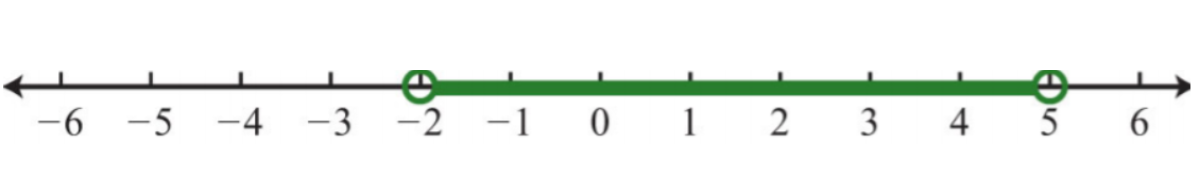Figure 2.7.25

3. $$(−5, 20]$$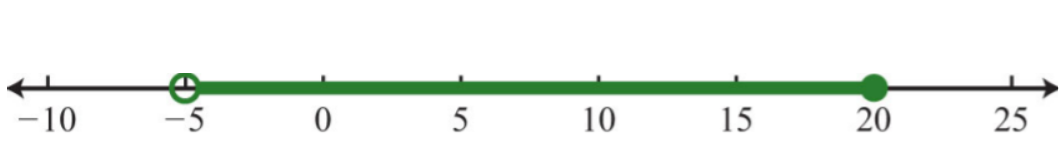Figure 2.7.26

5. $$(10, 40]$$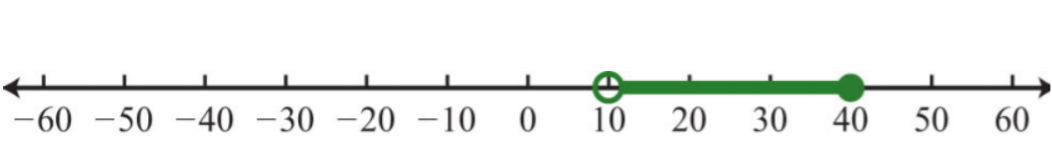Figure 2.7.27

7. $$(0, 50]$$Figure 2.7.28

9. $$(−\frac{5}{8}, \frac{1}{8})$$Figure 2.7.29

11. $$[−1, 1\frac{1}{2})$$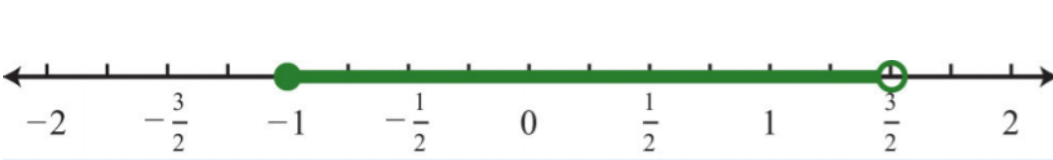Figure 2.7.30

13. $$(−∞, −3)∪(3, ∞)$$Figure 2.7.31

15. $$(−∞, 0]∪(10, ∞)$$Figure 2.7.32

17. $$(−∞, −\frac{2}{3})∪(\frac{1}{3}, ∞)$$Figure 2.7.33

19. $$R$$Figure 2.7.34

21. $$R$$Figure 2.7.35

23. $$(−∞, 2)$$Figure 2.7.36

25. $$(0, ∞)$$Figure 2.7.37

27. $$(−2, 3)$$Figure 2.7.38

29. $$[−5, −1]$$Figure 2.7.39

31. $$∅$$Figure 2.7.40

33. $$\{0\}$$Figure 2.7.41

35. $$(0, ∞)$$Figure 2.7.42

Exercise $$\PageIndex{3}$$ Interval Notation

Determine the inequality given the answers expressed in interval notation.

1. $$(−∞, 7]$$
2. $$(−4, ∞)$$
3. $$[−\frac{1}{2}, ∞)$$
4. $$(−∞, −3)$$
5. $$(−8, 10]$$
6. $$(−20, 0]$$
7. $$(−14, −2)$$
8. $$[\frac{2}{3}, \frac{4}{3}]$$
9. $$(−\frac{3}{4}, \frac{1}{2})$$
10. $$(−∞, −8)$$
11. $$(8, ∞)$$
12. $$(−∞, 4)∪[8, ∞)$$
13. $$(−∞, −2]∪[0, ∞)$$
14. $$(−∞, −5]∪(5, ∞)$$
15. $$(−∞, 0)∪(2, ∞)$$
16. $$(−∞, −15)∪(−5, ∞)$$

1. $$x\leq 7$$

3. $$x≥−\frac{1}{2}$$

5. $$−8<x\leq 10$$

7. $$−14<x<-2$$

9. $$-\frac{3}{4}<x<\frac{1}{2}$$

11. $$x>8$$

13. $$x≤−2$$ or $$x≥0$$

15. $$x<0$$ or $$x>2$$

Exercise $$\PageIndex{4}$$ Interval Notation

Write an equivalent inequality.

1. All real numbers less than $$27$$.
2. All real numbers less than or equal to zero.
3. All real numbers greater than $$5$$.
4. All real numbers greater than or equal to $$−8$$.
5. All real numbers strictly between $$−6$$ and $$6$$.
6. All real numbers strictly between $$−80$$ and $$0$$.

1. $$x<27$$

3. $$x>5$$

5. $$-6<x<6$$

Exercise $$\PageIndex{5}$$ Discussion Board Topics

1. Compare interval notation with set-builder notation. Share an example of a set described using both systems.
2. Explain why we do not use a bracket in interval notation when infinity is an endpoint.
3. Research and discuss the different compound inequalities, particularly unions and intersections.
4. Research and discuss the history of infinity.
5. Research and discuss the contributions of Georg Cantor.
6. What is a Venn diagram? Explain and post an example.